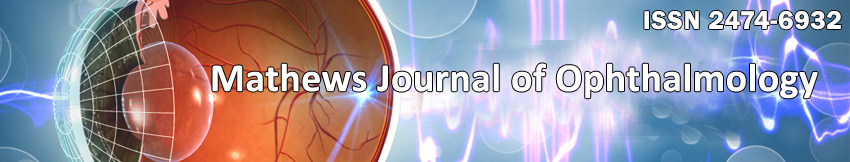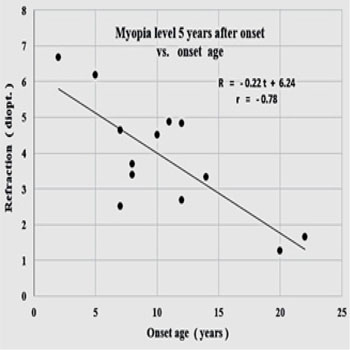Mini Review

#### Juvenile Myopia. Predicting the Progression Rate

###### Peter R. Greene1*, Antonio Medina2

1B.G.K.T. Consulting Ltd. Bioengineering Huntington, New York.

2Massachusetts Institute of Technology, Research Laboratory of Electronics, Cambridge, Mass, 02139.

Corresponding Author: Peter R Greene, B.G.K.T. Consulting Ltd. Bioengineering Huntington, New York, 11743, Tel: +1 631 935 56 66; E-Mail:prgreeneBGKT@gmail.com

• Received Date: 24 Dec 2016   Accepted Date: 09 Jan 2017   Published Date: 13 Jan 2017
• Copyright © 2017 Greene PR

Citation: Greene PR and Medina A. (2017). Juvenile Myopia. Predicting the Progression Rate. M J Opht. 2(1): 012.

###### ABSTRACT

Regression plots are generated showing the strong correlation of myopia onset age with its progression rate, r = -0.77, p< 0.0025, and strong correlation of accumulated myopia 5 years after onset, r = -0.78, p < 0.001. Theory is confirmed, with all subjects showing excellent correlation coefficients, = -0.971, p < 0.005. Myopia progression rates vary from 0.2 to 1.0 diopters (D) per year from one individual to the next. Age of onset for myopia is 10.6 yrs. +/-5.4 yrs. Initial refraction at onset is -1.14 D +/-0.55 D. Practical applications of this work include delaying correction for myopes, and juvenile susceptibility to myopia at various age levels. Feedback Theory predicts the results.

###### KEYWORDS

Emmetropia; Emmetropization; Myopia; Susceptibility; Feedback Model; Under Correction.

INTRODUCTION

TMyopia has an estimated prevalence of 41% among adults in the United States, and the myopia prevalence is increasing Vitale et al. . Among Asian populations, the prevalence is even higher with rates as high as 60% among adolescents age 11 to 17 yrs in China [2-4].
The course of myopia typically follows a pattern that begins with an initial emmetropic phase, followed by a myopic onset that usually occurs in the early school years, which is followed by a myopic depression that tends to stabilize in the mid to late teenage years . These are general trends, early or late onset myopia is also possible and a more modest progression may occur during early adulthood before fully stabilizing.Figure 1a: The transfer function that describes emmetropization is F(s) = 1/ (ks+1), where k is the time constant of each individual and s is the complex variable. G(s) = 1/ks is the forward function of the transfer function.

Several facts are established that allow an understanding of refraction development of the eye as a system. Emmetropization and refractive development is a feedback process in humans [6-11]. There is now considerable evidence showing that there is feedback control of emmetropization. Emmetropic or uncorrected eyes follow an exponential development of refractive error in humans, as shown in Figure 1a. An exponential development is the response of a first-order feedback system to a constant-level step input signal [9, 10]. Such a 1st order model will quantitatively model not only the mechanism of emmetropization, but also the effect of lenses [6, 7].
The refractive state of the eye is alterable with external stimuli, including lid suture. (Meyer et al. ; Raviola & Wiesel ; Greene & Guyton ; Hoyt at al. ; Wallman & Winawer ). Medina & Fariza  showed that corrective lenses applied to the eye are step input stimuli to the emmetropization system and that the response of a first order system (Feedback Theory) to an input determined by the power of the corrective lenses fits refraction data from ametropic subjects that wore those lenses .
Medina [9, 10] showed that a myope that is fully corrected continuously places the emmetropization feedback system in an open loop condition, as shown in Figure 1b. Continuous correction alters the feedback loop rendering it inoperative. He also found that refractive data from the eyes of 13 myopic subjects followed straight lines as predicted by Feedback Theory, Figure 2. Fledelius presents distribution diagrams of onset age for myopia from age 6 to 27 (N=184 subjects), which can be compared to Figure 2 here .Figure 1b: Open loop transfer function of the feedback system of transfer function 1/ (ks+1). Broken lines denote the loop is open. This open loop function describes continuous correction or visual form deprivation (constant error). The variables are t, time and s, complex variable.

Figure 2 shows the refractive errors of right eyes of 13 myopic human subjects versus age (symbols) and linear prediction of open loop system (solid lines). Myopia rates range from -0.2 to -1.0 D/yr. Correlation coefficients range from -0.907 to -0.998, with a mean of = -0.971, p< 0.005. Three other similar studies from the literature report essentially the same myopia progression rates, Oakley & Young , Goss , and Gwiazda et al. [20, 21] for young individuals. Goss & Rainey et al.  report myopia rates (N=42 subjects) of -0.72 D/yr during the school year, compared with -0.39 D/yr during the summer months.Figure 2: Refractive errors of right eyes of 13 myopic human subjects versus age (symbols) and linear prediction of open loop system (R/k, solid lines), from Medina [9, 10].

MATERIALS AND METHODS

The clinical techniques and data reduction procedures employed to generate Figure 2 are described in Medina et al. [9, 10]. These straight-line trajectories are typical of juvenile progressive myopes. The slope of these lines allows us to correlate average myopia progression rate versus onset age t1 (Figure 3a). Another benchmark indicator of myopia progression is the total myopia accumulated 5 years after onset, Figure 3b, graphed versus initial onset age. Correlation coefficient r and significance level p quantify the accuracy of the regression.Figure 3a: Myopia progression rate vs. age of onset, r = - 0.77, p < 0.0025, < R’> = - 0.55 D/yr +/- 0.27 D/yr.Figure 3b: Myopia level 5 years after onset vs. onset age, r = - 0.78, p<0.001, = - 4.51 D. +/-1.28 D.

RESULTS

Figures 3a and 3b show that myopia rate and total acquired myopia 5 years after onset are strongly correlated with onset age, r = -0.77, p< 0.0025, and r = -0.78, p< 0.001 respectively. Average myopia onset age is = 10.6y +/- 5.4y (N=13). Initial refraction at onset is on average = -1.14D. +/- 0.55D, correlation with age of onset r = -0.30, p< 0.25.

DISCUSSION

These results indicate that the juvenile myope is twice as susceptible to myopia progression at age 5 as at age 15 (-0.8 D/yr. versus -0.4 D/yr.) and twice as susceptible at age 10 as at age 18 (-0.6 D/yr. vs. -0.3 D/yr.). Likewise, at onset, the initial refraction at age 5 is expected to be 50% greater than at age 18, (-1.3 D. vs. -0.9 D. respectively). Confirming these results it was recently found that the risk of high myopia can be predicted based on age of onset of myopia . Feedback Theory predicts the results as it provides that the slope of the progression line is proportional to the myopia at onset.

CONCLUSION

Feedback Theory and the observations described here have some practical implications concerning the correction or under correction of myopes [24-28].

REFERENCES

###### Citation:
Greene PR and Medina A. (2017). Juvenile Myopia. Predicting the Progression Rate. M J Opht. 2(1): 012.